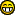News: Support us via Flattr FLATTR Link## The All New FractalForums is now in Public Beta Testing! Visit FractalForums.org and check it out!

 Pages:    Go DownAuthor Topic: Having fun with scilab and the mandelbrot set  (Read 4931 times) Description: 0 Members and 1 Guest are viewing this topic.
ker2x
Fractal MolossusPosts: 795« on: February 22, 2012, 10:06:57 PM »

I'm learning scilab, i don't understand everything in the code below.
But i find interesting the way to compute the mandelbrot setIf you don't know scilab, it's free, opensource, powerful, multiplateform, slowhttp://www.scilab.org/

Code:
// In the row matrix J, we store the indices of the points in the Mandelbrot set.
// Initially, the matrix J contains the indices from 1 to xsize*ysize,
// meaning that all the points are in the set.
// During the computation, we are going to remove points from the set,
// by setting the corresponding entry of J to zero.
// In the current iteration, the set of indices of points which are in the set
// is therefore computed with the L = J(J>0) statement.
// Hence, when the algorithm progresses, there are less and less points in the
// set so that the matrix L has a decreasing number of entries.
// The operations are done only on the entries defined in L.
// This makes the algorithm faster and faster.

function R = computeMandelbrotVect(xsize,ysize,nmax,xmin,xmax,ymin,ymax)
//Init matrix
xvect = linspace( xmin, xmax, xsize );
yvect = linspace( ymin, ymax, ysize );
[X,Y]=meshgrid(xvect,yvect);

//Inits values
Z = zeros(xsize,ysize);    //The Z of Z = Z²+C
R = -ones(xsize,ysize);
W = zeros(xsize,ysize);    // Will store the distance from 0,0

C=X+%i*Y;                  //Set the value of C
J = 1:xsize*ysize;
for k=0:nmax               // 0 to max iteration
L = J(J>0);
Z(L) = Z(L).^2+C(L);       // Z = Z² + C
W(L) = abs(Z(L));          // Compute Distance
M = find(W(L)>2);          // Find if Distance > 2
R(L(M)) = k;
J(L(M)) = 0;
end
R = R';
// The maximum number of colors
CMAX = 1000;
f=gcf();
f.color_map = jetcolormap(CMAX);
D = R;
k = find(R<>-1);
D(k) = floor(R(k)/max(R(k))*CMAX);
k = find(R==-1);
D(k) = CMAX;
Matplot(D);
f.children.isoview="on";
f.children.axes_visible=["off" "off" "off"];
endfunction

stacksize("max");
xsize = 200;
ysize = 200;
nmax = 1000;
xmin = -2.0;
xmax = 1.0;
ymin = -1.0;
ymax = 1.0;

mprintf("begin...");
computeMandelbrotVect(xsize,ysize,nmax,xmin,xmax,ymin,ymax);
mprintf("done!");Logged

often times... there are other approaches which are kinda crappy until you put them in the context of parallel machines
(en) http://www.blog-gpgpu.com/ , (fr) http://www.keru.org/ ,
ker2x
Fractal MolossusPosts: 795« Reply #1 on: February 22, 2012, 10:09:32 PM »

this part
Code:
C=X+%i*Y;

is like
Code:
C=complex(X,Y)Logged

often times... there are other approaches which are kinda crappy until you put them in the context of parallel machines
(en) http://www.blog-gpgpu.com/ , (fr) http://www.keru.org/ ,
 Related Topics Subject Started by Replies Views Last post3D Mandelbrot Movies Showcase (Rate My Movie) David Makin 0 2194December 09, 2006, 11:38:14 PM by David MakinMandelbrot 3d Mutatorkammer Gallery cKleinhuis 2 2086March 19, 2008, 05:45:40 PM by GFWorld3d mandelbrot Selfmade lycium 0 4095April 27, 2008, 09:16:43 PM by lyciumThe Mandelbrot Set Introduction to Fractals and Related Links « 1 2 » billboll 25 43282February 15, 2013, 02:23:51 AM by KingOfOwlsScilab vs Mathlab vs Mathematica ? General Discussion ker2x 8 7934June 17, 2010, 04:23:20 PM by hobold